# [Algorithms I] Week 2-1 Stacks and Queues

[TOC]

fundamental data types: stacks and queues operations: insert, remove, test empy, iterate,...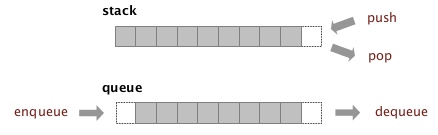module programming: seperate interface and implementation

# 1. Stacks

ex. a stack of strings

• API:

public interface StackoOfStrings{ void push(String item); String pop(); boolean isEmpty(); //int size(); }

### implementation 1: using a linkedlist

insert/remove from the top of the linkedlist

• inner class

```class ListNode{
String item;
ListNode next;
}
```
• implementation

```public class LinkedStackOfStrings implements StackoOfStrings{
class Node{
String item;
Node next;
Node(String item, ListNode nxt){...}
}
private Node first;

public LinkedStackOfStrings(){
first = null;
}
public void push(String item){
Node nd = new Node(item,first);
first = nd;
}
public String pop(){
String firstItem = first.item;
first = first.next;
return firstItem;
}
public boolean isEmpty(){
return first==null;
}
}
```
• complexity: const time for every operation

### array implementation

• use array (of length N) to store items → defect: stack has limited capacity
• keep a pointer *top: *pointing to the next empty space to push (top 的定义很重要)

• problems of the array implementation:

1. underflow: pop from an empty stack
2. overflow: size larger than capacity ⇒ resizing
3. loitering: holding a ref to an obj which is no longer needed: ex. `return s[top--]` java system will not know that s[top] is no longer needed ⇒ have to clear it explicitely ⇒

String item = s[top--]; s[top]=null; return item

• implementation (containg resizing array operations)

```public class ArrayStackOfStrings implements StackOfStrings{
private String[] s;
private int top=0;
public ArrayStackOfStrings(){// to be tuned
s = new String;//initial capacity=1
}
public boolean isEmpty(){
return top==0;
}
private vois resize(int capacity){//helper functoin
String[] s2 = new String[capacity];
for(int i=0;i<top;i++)
s2 = s[i];
s = s2;
}
public void push(String item){
if(top==s.length)//doubling size
resize(s.lenth*2);
s[top++]=item;
}
public String pop(){
String item = s[--top];//NOT top--!
s[top]=null;
if(top>0 && top==s.length/4) //top>0 is necessary
resize(s.length/2);
return item;
}
}
```

# 2. Resizing Arrays

resolving the overflow pb: grow and shrink the array → need to copy all items when changing array size ⇒ pb: ensure that sizing happens infrequently

### resizing strategy

• repeated doubling:

(initial capacity=1) when array is full, double the size

• amortized complexity for inserting N:

N+(2+4+8+...+N) ~3N

• shrinking array

⇒ shrink the array by half when array is 1/4 full not half full → thrashing will happen if push-pop-push-pop when array is full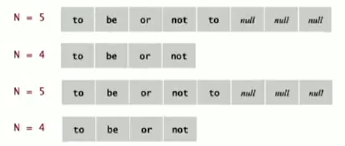• [invariant]: array always 20%~100% full
• complexity:

in an amortized sense, will be constant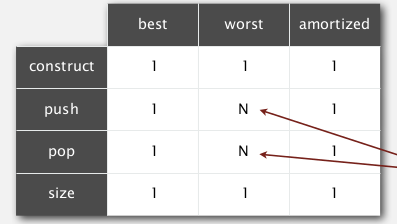proposition: from empty stack, M operations of push/pop taked time propotional to M

### comparison: resizable array vs linkedlist

• linkedlist implementation:

operations takes const time even in worst time extra time and space for dealing with linkes

• resizing array implementation:

operation taked const amortized time but in worst case takes linear time (ex. to be evited for critical systems) less wasted space

## 3. Queues

FIFO data structure API

```public interface QueueOfStrings{
void enqueue(String item);
String dequeue();
boolean isEmpty();
//int size();
}
```

### linked list implementation

maintain `first` and `last` node pointers: pointing to 2 points of queue (`first` for dequeue, `last` for enqueue )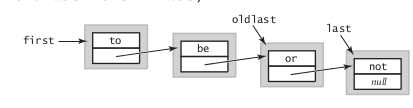take care of corner cases: - empty queue: first is null (and last is also null) - just one item in queue: first and last point to the same node (总之first和last的定义很重要)

```    public class LinkedQueueOfStrings implements QueueOfStrings{
class Node{...  }
private Node first,last;
public LinkedQueueOfStrings(){
first = null;
}
public void enqueue(String item){//same as push
Node nd = new Node(item,null);
if(isEmpty()){
last = nd;
first = last;
}
else{
last.next = nd;
last = nd;
}
}
public String dequeue(){//same as pop in stack
String firstItem = first.item;
first = first.next;
if(isEmpty())
last=null;
return firstItem;
}
public boolean isEmpty(){
return first==null;
}
}
```

### resizing array implementation

maintain `head` and `tail`: `head` is the queue head, `tail` is the next empty position for the next element to enqueue → trick: head and tail should take mod capacity + resizing array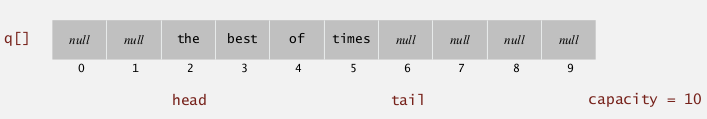```    public class ArrayQueueOfStrings implements QueueOfStrings{
private String[] q;
private head=0,tail=0;
public LinkedQueueOfStrings(){
q = new String;//init capacity
}
public boolean isEmpty(){
return head==tail;
}
private void resize(newsz){
q2 = new String[newsz];
int i = head,j=0;
while(i!=tail){
q2[j++] = q[i];
i=(i+1)%q.length;
}
q = q2;
head=0;
tail=j;
}
public void enqueue(String item){
if( (tail+1)%q.length==head )
resize(q.length*2);
q[tail] = item;
tail = (tail+1)%q.length;
}
public String dequeue(){
String firstItem = q[head];
head = (head+1)%q.length;
int sz = (tail-head)%q.length;
if(sz>0 && sz==q.length/4)
resize(s.length/2);
return firstItem;
}
}
```

# 4. Generics

queues/stacks for other types of data ⇒ generics 泛型(java 1.5 才引进泛型机制...) use type paramater→ avoid casting, and discover type mismatch errors at compile time

```    public interface Stack<Item>{
public void push(Item item);
public Item pop();
public boolean isEmpty();
}
```

### a pb with array implementation

java不支持创立泛型数组 generic array creation is not allowed. 不可以new 一个泛型数组! `s = new Item[capacity];`会报错
⇒ use an ugly cast: `s = (Item[]) new Object[capacity];` (will get warning: "unchecked cast" → java被黑了... )

### autoboxing for primitive types

each primitive type has a wrapper class ex. int ↔ Integer autoboxing: automatic cast between a primitive type and its wrapper class.(syntactic sugar 语法糖 i.e. 对语言功能没有影响只是方便使用)
btw: https://zh.wikipedia.org/wiki/%E8%AF%AD%E6%B3%95%E7%B3%96 (居然还有语法盐和语法糖精......)

# 5. Iterators

### Interface

support iteration over stacks and queues, without revealing the internal representation of stack/queue ⇒ implement the `Iterable` interface

• `Iterable` interface: can return an *Iterator *

```public interface Iterable{
Iterator<Item> iterator();
}
```
• Iterator interface: hasNext() and next() interface

```public interface Iterator<Item>{
boolean hasNext();
Item next();
void remove();//optional, bad practice to use it
}
```
• to make a data structure Interable → elegant client code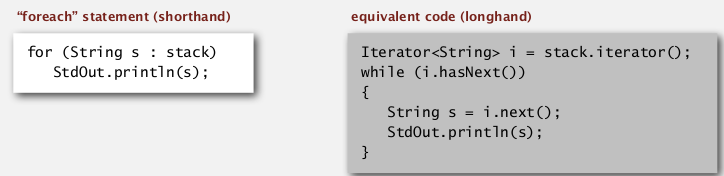how-to:

1. implement `Iterable` interface
2. write a private inner class XXIterator that implment the `Iterator` interface.

ex.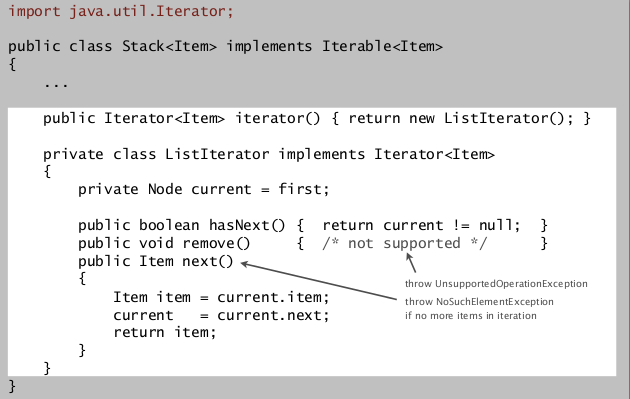### Bag data structure

Supports adding and iterating through without caring about the order.
API:

```    public class<Item> Bag implements Iterable<Item>{
public void add(Item);
int size();
}
```

can be implemented by stack or queue(without pop/dequeue)

# 6. Applications

### Java collections library

List interface: `java.util.List`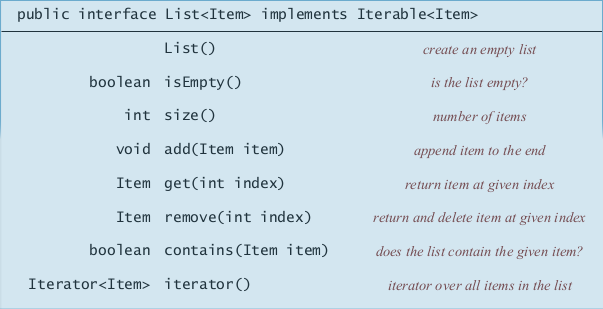implementations: `ArrayList`, `LinkedList`.

• pb with the java's implementation of stacks and queues:

`Stack` class also implements List interface (`get()`, `remove()`, `contains()` are implemented);
`Queue` is an interface rather than a class...
poorly designed API

### Stacks applications

• function calls: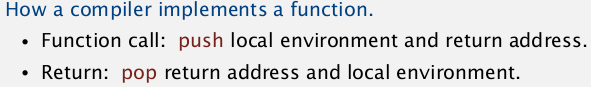recursion: can always use an explicit stack to remove recursion

• arithemic evaluation (Dijkstra)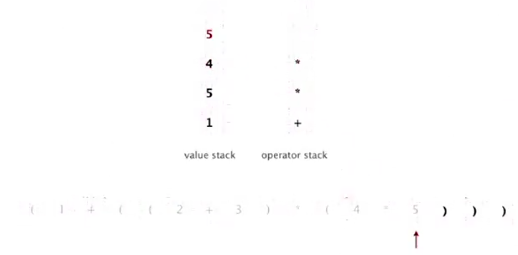⇒ 后缀表达式, 逆波兰式......

#### Part 4 of series «Algorithms Princeton MOOC I»：

comments powered by Disqus